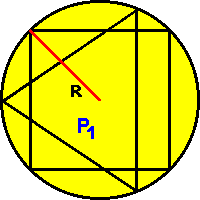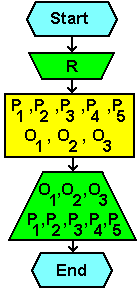# 6.1.3. Surface of trigonometric figures1. phase
Calculate the perimeter and surface of figures.

2. phase
Calculate the surface and of perimeter a circle with radius R, and the squares and equilateral triangles blend in a circle with corners that lie at the edge of the circle.
Calculate the surface of a circle slices belonging to the square and triangle.

3. phase
Mathematical constants, and expressions for the specified task are:PI = 3.1415927 -------------------- P1 = R2•PI P2 = 2•R2 P3 = 2•R2•sin3(PI / 3) P4 = (P1 - P2) / 4 P5 = (P1 - P3) / 3 -------------------- O1 = 2•R•PI O2 = 4•2(1 / 2)•R O3 = 6•R•sin(PI / 3)

4. phase
Flowchart:Citing of this page: Radic, Drago. " IT - Informatics Alphabet " Split-Croatia. {Date of access}. . Copyright © by Drago Radic. All rights reserved. | Disclaimer